# Predator-Prey period

The Lotka-Volterra equations are a system of nonlinear differential equations for modeling a predator-prey ecosystem. After a suitable change of units the equations can be written in the formwhere ab = 1. Here x(t) is the population of prey at time t and y(t) is the population of predators. For example, maybe x represents rabbits and y represents foxes, or x represents Eloi and y represents Morlocks.

It is well known that the Lotka-Volterra equations have periodic solutions. It is not as well known that you can compute the period of a solution without having to first solve the system of equations.

This post will show how to compute the period of the system. First we’ll find the period by solving the equations for a few different initial conditions, then we’ll show how to directly compute the period from the system parameters.

## Phase plot

Here is a plot of (x(t), y(t)) showing that the solutions are periodic.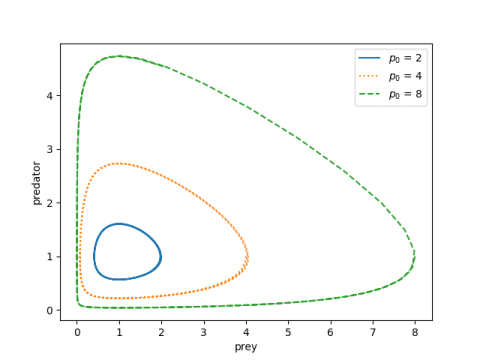And here’s the Python code that made the plot above.

    import matplotlib.pyplot as plt
import numpy as np
from scipy.integrate import solve_ivp

# Lotka-Volterra equations
def lv(t, z, a, b):
x, y = z
return [a*x*(y-1), -b*y*(x-1)]

begin, end = 0, 20
t = np.linspace(begin, end, 300)

styles = ["-", ":", "--"]
prey_init = [2, 4, 8]
a = 1.5
b = 1/a

for style, p0 in zip(styles, prey_init):
sol = solve_ivp(lv, [begin, end], [p0, 1], t_eval=t, args=(a, b))
plt.plot(sol.y, sol.y, style)

plt.xlabel("prey")
plt.ylabel("preditor")
plt.legend([f"$p_0$ = {p0}" for p0 in prey_init])


Note that the derivative of x is zero when y = 1. Since our initial condition sets y(0) = 1, we’re specifying the maximum value of x. (If our initial values of x were less than 1, we’d be specifying the minimum of x.)

## Time plot

The components x and y have the same period, and that period depends on a and b, and on the initial conditions. The plot below shows how the period increases with x(0), which as we noted above is the maximum value of x.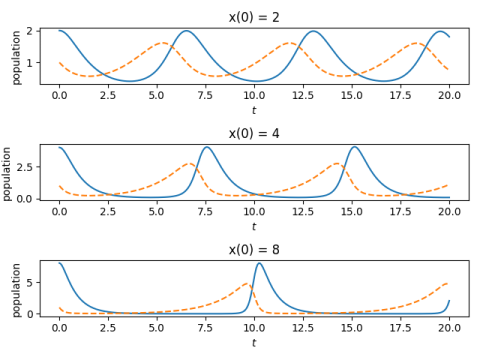And here’s the code that made the plot.

    fig, ax = plt.subplots(3, 1)
for i in range(3):
sol = solve_ivp(lv, [begin, end], [prey_init[i], 1], t_eval=t, args=(a, b))
ax[i].plot(t, sol.y, "-")
ax[i].plot(t, sol.y, "--")
ax[i].set_xlabel("$t$")
ax[i].set_ylabel("population")
ax[i].set_title(f"x(0) = {prey_init[i]}")
plt.tight_layout()


## Finding the period

In  the author develops a way to compute the period of the system as a function of its parameters without solving the differential equations.

First, calculate an invariant h of the system:Since this is an invariant we can evaluate it anywhere, so we evaluate it at the initial conditions.

Then the period only depends on a and h. (Recall we said we can scale the equations so that ab = 1, so a and b are not independent parameters.)

If h is not too large, we can compute the approximate period using an asymptotic series.where σ = (a + b)/2 = (a² + 1)/2a.

We use this to find the periods for the example above.

    def find_h(a, x0, y0):
b = 1/a
return b*(x0 - np.log(x0) - 1) + a*(y0 - np.log(y0) - 1)

def P(h, a):
sigma = 0.5*(a + 1/a)
s = 1 + sigma*h/6 + sigma**2*h**2/144
return 2*np.pi*s

print([P(find_h(1.5, p0, 1), 1.5) for p0 in [2, 4, 8]])


This predicts periods of roughly 6.5, 7.5, and 10.5, which is what we see in the plot above.

When h is larger, the period can be calculated by numerically evaluating an integral given in .

## Related posts

 Jörg Waldvogel. The Period in the Volterra-Lotka Predator-Prey Model. SIAM Journal on Numerical Analysis, Dec., 1983, Vol. 20, No. 6, pp. 1264-1272

# Oscillatory differential equations

The solution to a differential equation is called oscillatory if its set of zeros is unbounded. This does not necessarily mean that the solution is periodic, but that it crosses the horizontal axis infinitely often.

Fowler  studied the following differential equation demonstrates both oscillatory and nonoscillatory behavior.

x” + tσ |x|γ sgn x = 0

He proved that

1. If σ + 2 ≥ 0 then all solutions oscillate.
2. If σ + (γ + 3)/2 < 0 then no solutions oscillate.
3. If neither (1) nor (2) holds then some solutions oscillate and some do not.

The edge case is σ = -2 and γ = 1. This satisfies condition (1) above. A slight decrease in σ will push the equation into condition (2). And a simultaneous decrease in σ and increase in γ can put it in condition (3).

It turns out that the edge case can be solved in closed form. The equation is linear because γ = 1 and solutions are given by

ct sin(φ + √3 log(t) / 2).

The solutions oscillate because the argument to sine is an unbounded function of t, and so it runs across multiples of π infinitely often. The distance between zero crossings increases exponentially with time because the logarithms of the crossings are evenly spaced.

This post took a different turn after I started writing it. My original intention was to solve the differential equation numerically and say “See: when you change the parameters slightly you get what the theorem says.” But that didn’t work out.

First I tried numerically computing the solution above with σ = -2 and γ = 1, but I didn’t see oscillations. I tried making σ a little larger, but still didn’t see oscillations. When I increased σ more I could see the oscillations.

I was able to go back and see the oscillations with σ = -2 and γ = 1 by tweaking the parameters in my ODE solver. It’s best practice to tweak your parameters even if everything looks right, just to make sure your solution is robust to algorithm changes. Maybe I would have done that, but since I already knew the analytic solution, I knew to tweak the parameters until I saw the right behavior.

Without some theory as a guide, it would be difficult to determine from numerical solutions alone whether a solution oscillates. If you see oscillations, then they’re probably real (unless your numerical method is unstable), but if you don’t see oscillations, how do you know you won’t see them if you look further out? How much further out should you look? In a practical application, the context of your problem will tell you how far out to look.

My intention was to recommend the equation above as an illustration for an introductory DE class because it demonstrates the important fact that small changes to an equation can qualitatively change the behavior of the solutions. This is especially true for nonlinear DEs, which the above equation is when γ ≠ 1. It could still be used for that purpose, but it would make a better demonstration than homework exercise. The instructor could tune the DE solver to make sure the solutions are qualitatively correct.

I recommend the equation as an illustration, but for a course covering numerical solutions to DEs. It illustrates a different point than I first intended, namely the potentially finicky behavior of software for solving differential equations.

There are a couple morals to this story. One is that numerical methods have not eliminated the need for theory. The ideal is to combine analytic and numerical methods. Analytic methods point out qualitative behavior that might be hard to discover numerically. And analytic methods are not likely to produce closed-form solutions for nonlinear DEs.

Another moral is that it’s best to twiddle the parameters to your numerical method to make sure the solution doesn’t qualitatively change. If you’ve adequately computed a solution, computing it again with smaller integration steps shouldn’t make much difference. But if you do see a substantial difference, your first solution probably wasn’t as good as you thought.

## Related posts

 R. H. Fowler. Further studies of Emden’s and similar differential equations. Quarterly Journal of Mathematics. 2 (1931), pp. 259–288.

# Laplacian in various coordinate systems

The recent post on the wave equation on a disk showed that the Laplace operator has a different form in polar coordinates than it does in Cartesian coordinates. In general, the Laplacian is not simply the sum of the second derivatives with respect to each variable.

Mathematica has a function, unsurprisingly called Laplacian, that will compute the Laplacian of a given function in a given coordinate system. If you give it a specific function, it will compute the Laplacian of that function. But you can also give it a general function to find a general formula.

For example,

    Simplify[Laplacian[f[r, θ], {r, θ}, "Polar"]]

returnsThis is not immediately recognizable as the Laplacian from this postbecause Mathematica is using multi-index notation, which is a little cumbersome for simple cases, but much easier to use than classical notation when things get more complicated. The superscript (0,2), for example, means do not differentiate with respect to the first variable and differentiate twice with respect to the second variable. In other words, take the second derivative with respect to θ.

Here’s a more complicated example with oblate spheroidal coordinates. Such coordinates come in handy when you need to account for the fact that our planet is not exactly spherical but is more like an oblate spheroid.

    Simplify[Laplacian[f[ξ, η, φ], {ξ, η, φ}, "OblateSpheroidal"]]

the result isn’t pretty.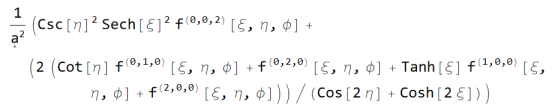I tried using TeXForm and editing it into something readable, but after spending too much time on this I gave up and took a screenshot. But as ugly as the output is, it would be uglier (and error prone) to do by hand.

Mathematica supports the following 12 coordinate systems in addition to Cartesian coordinates:

• Cylindrical
• Bipolar cylindrical
• Elliptic cylindrical
• Parabolic cylindrical
• Circular parabolic
• Confocal paraboloidal
• Spherical
• Bispherical
• Oblate spheroidal
• Prolate spheroidal
• Conical
• Toroidal

These are all orthogonal, meaning that surfaces where one variable is held constant meet at right angles. Most curvilinear coordinate systems used in practice are orthogonal because this simplifies a lot of things.

Laplace’s equation is separable in Stäckel coordinate systems. These are all these coordinate systems except for toroidal coordinates. And in fact Stäckel coordinates are the only coordinate systems in which Laplace’s equation is separable.

It’s often the case that Laplace’s equation is separable in orthogonal coordinate systems, but not always. I don’t have a good explanation for why toroidal coordinates are an exception.

If you’d like a reference for this sort of thing, Wolfram Neutsch’s tome Coordinates is encyclopedic. However, it’s expensive new and hard to find used.

# Vibrating circular membranesThis post will tie together many things I’ve blogged about before. The previous post justified separation of variables. This post will illustrate separation of variables.

Also, this post will show why you might care about Bessel functions and their zeros. I’ve written about Bessel functions before, and said that Bessel functions are to polar coordinates what sines and cosines are to rectangular coordinates. This post will make this analogy more concrete.

## Separation of variables

The separation of variables technique is typically presented in three contexts in introductory courses on differential equations:

1. One-dimensional heat equation
2. One-dimensional wave equation
3. Two-dimensional (rectangular) Laplace equation

My initial motivation for writing this post was to illustrate separation of variables outside the most common examples. Separation of variables requires PDEs to have a special form, but not as special as the examples above might imply. A secondary motivation was to show Bessel functions in action.

Suppose you have a thin membrane, like a drum head, and you want to model its vibrations. By “thin” I mean that the membrane is sufficiently thin that we can adequately model it as a two-dimensional surface bobbing up and down in three dimensions. It’s not so thick that we need to model the material in more detail.

Let u(x, y, t) be the height of the membrane at location (x, y) and time t. The wave equation is a PDE modeling the motion of the membrane bywhere Δ is the Laplacian operator. In rectangular coordinates the Laplacian is given byWe’re interested in a circular membrane, and so things will be much easier if we work in polar coordinates. In polar coordinates the Laplacian is given byWe will assume that our boundary conditions and initial conditions are radially symmetric, and so our solution will be radially symmetric, i.e. derivatives with respect to θ are zero. And in our case the wave equation simplifies to## Boundary and initial conditions

Let a be the radius of our membrane. We will assume our membrane is clamped down on its boundary, like a drum head, which means we have the boundary conditionfor all t ≥ 0. We assume the initial displacement and initial velocity of the membrane are given byfor all r between 0 and a.

## Separating variables

Now we get down to separation of variables. Because we’re assuming radial symmetry, we’re down to a function of two variables: r for the distance from the center and t for time. We assumeand stick it into the wave equation. A little calculation shows thatThe left side is a function of t alone, and the right side is a function of r alone. The only way this can be true for all t and all r is for both sides to be constant. Call this constant -λ². Why? Because looking ahead a little we find that this will make things easier shortly.

Separation of variables allowed us to reduce our PDE to the following pair of ODEs.The solutions to the equation for R are linear combinations of the Bessel functions J0r) and Y0r) .

And the solutions to the equation for T are linear combinations of the trig functions cos(cλt) and sin(cλt).

The boundary condition u(a, t) = 0 implies the boundary condition R(a) = 0. This implies that λa must be a zero of our Bessel function, and that all the Y0 terms drop out. This means that our solution iswhereHere αn are the zeros of of the Bessel function J0.

The coefficients An and Bn are determined by the initial conditions. Specifically, you can show thatThe function J1 in the expression for the coefficients is another Bessel function.

The functions J0 and J1 are so important in applications that even the otherwise minimalist Unix calculator bc includes these functions. (As much as I appreciate Bessel functions, this still seems strange to me.) And you can find functions for zeros of Bessel functions in many libraries, such as scipy.special.jn_zeros in Python.

## Related posts

 This is why introductory courses are unlikely to include an example in polar coordinates. Separation of variables itself is no harder in polar coordinates than in rectangular coordinates, and it shows the versatility of the method to apply it in a different setting.

But the resulting ODEs have Bessel functions for solutions, and it’s understandable that an introductory course might not want to go down this rabbit trail, especially since PDEs are usually introduced at the end of a differential equation class when professors are rushed and students are tired.

# Justifying separation of variables

The separation of variables technique for solving partial differential equations looks like a magic trick the first time you see it. The lecturer, or author if you’re more self-taught, makes an audacious assumption, like pulling a rabbit out of a hat, and it works.

For example, you might first see the heat equation

ut = c² uxx.

The professor asks you to assume the solution has the form

u(x, t) = X(x) T(t).

i.e. the solution can be separated into the product of a function of x alone and a function of t alone.

Following that you might see Laplace’s equation on a rectangle

uxx + uyy = 0

with the analogous assumption that

u(x, y) = X(x) Y(y),

i.e. the product of a function of x alone and a function of y alone.

There are several possible responses to this assumption.

1. Whatever you say, doc.
2. How can you assume that?
3. How do you know you’re not missing any possibilities?
4. What made someone think to try this?

As with many things, separation of variables causes the most consternation for the moderately sophisticated students. The least sophisticated students are untroubled, and the most sophisticated student can supply their own justification (at least after the fact).

One response to question (2) is “Bear with me. I’ll show that this works.”

Another response would be “OK, how about assuming the solution is a sum of such functions. That’s a much larger space to look in. And besides, we are going to take sums of such solutions in a few minutes.” One could argue from functional analysis or approximation theory that the sums of separable functions are dense in reasonable space of functions .

This is a solid explanation, but it’s kind of anachronistic: most students see separation of variables long before they see functional analysis or approximation theory. But it would be a satisfying response for someone who is seeing all this for the second time. Maybe they were exposed to separation of variables as an undergraduate and now they’re taking a graduate course in PDEs. In an undergraduate class a professor could do a little foreshadowing, giving the students a taste of approximation theory.

Existence of solutions is easier to prove than uniqueness in this case because you can concretely construct a solution. This goes back to the “it works” justification. This argument deserves more respect than a sophomoric student might give it. Mathematics research is not nearly as deductive and mathematics education. You often have to make inspired guesses and then show that they work.

Addressing question (3) requires saying something about uniqueness. A professor could simply assert that there are uniqueness theorems that allow you to go from “I’ve found something that works” to “and so it must be the only thing that works.” Or one could sketch a uniqueness theorem. For example, you might apply a maximum principle to show that the difference between any two solutions is zero.

Question (4) is in some sense the most interesting question. It’s not a mathematical question per se but a question about how people do mathematics. I don’t know what was going through the mind of the first person to try separation of variables, or even who this person was. But a plausible line of thinking is that ordinary differential equations are easier than partial differential equations. How might you reduce a PDE to an ODE? Well, if the solution could be factored into functions of one variable, …

The next post will illustrate using separation of variables by solving the wave equation on a disk.

## Related posts

 Also, there’s the mind-blowing Kolmogorov-Arnol’d theorem. This theorem says any continuous function of several variables can be written as a sum of continuous separable functions. It doesn’t say you can make the functions in your sum smooth, but it suggests that sums of separable functions are more expressive than you might have imagined.

# Fourier, Gauss, and Heisenberg

Several weeks ago I wrote about the Fourier uncertainty principle which gives a lower bound on the product of the variance of a function f and the variance of its Fourier transform. This post expands on the earlier post by quoting some results from a recent paper .

## Gaussian density

The earlier post said that the inequality in the Fourier uncertainty principle is exact when f is proportional to a Gaussian probability density. G. H. Hardy proved this result in 1933 in the form of the following theorem.

Let f be a square-integrable function on the real line and assume f and its Fourier transform satisfy the following boundsfor some constant C. Then if ab > 1/4, then f = 0. And if ab = 1/4, f(x) = c exp(-ax²) for some constant c.

Let’s translate this into probability terms by settingNow Hardy’s theorem says that if f is bounded by a multiple of a Gaussian density with variance σ² and its Fourier transform is bounded by a multiple of a Gaussian density with variance τ², then the product of the two variances is no greater than 1. And if the product of the variances equals 1, then f is a multiple of a Gaussian density with variance σ².

## Heisenberg uncertainty

Theorem 3 in  says that if u(tx) is a solution to the free Schrödinger’s equationthen u at different points in time satisfies a theorem similar to Hardy’s theorem. In fact, the authors show that this theorem is equivalent to Hardy’s theorem.

Specifically, if u is a sufficiently smooth solution andthen αβ > (4T)-2 implies u(t, x) = 0, and αβ = (4T)-2 implies## Related posts

 Aingeru Fernández-Bertolin and Eugenia Malinnikova. Dynamical versions of Hardy’s uncertainty principle: A survey. Bulletin of the American Mathematical Society. DOI: https://doi.org/10.1090/bull/1729

# A stiffening spring

Imagine a spring with stiffness k1 attached to a ceiling and a mass m1 handing from the spring.

There’s a second spring attached to the first mass with stiffness k2 and a mass m2 handing from that.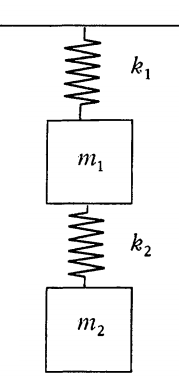The motion of the system is described by the pair of differential equationsIf the second spring were infinitely stiff, the two masses would be joined with a rigid rod, and so the system would act like a single mass m1 + m2 hanging from the first spring. This motion would be described by the single differential equationSo it’s not surprising that as k2 gets stiffer, the solution to the two-mass system converges to the solution to the system with a single combined mass. This is proven in .

However, what is missing from  is any visualization of how the solution to the two-mass system converges to that of the combined-mass system.

The plot below shows solutions for k2 equal to 10, 100, and 1000, and finally the system to the combined-mass system, labeled k2 = ∞. I used k1 = 1, m1 = 3, and m2 = 5.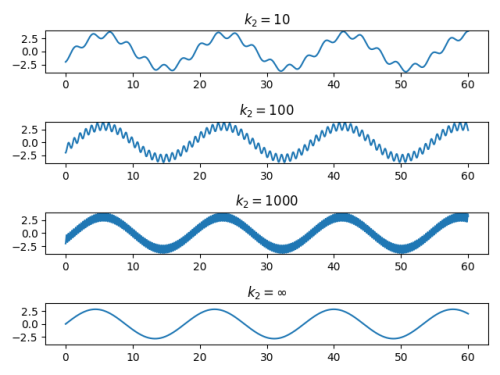The coupled-mass system has a high-frequency component due to the oscillation of the second mass relative to the first one.

The authors in  show that the amplitude of the high-frequency component decays as k2 goes to infinity, though this is not apparent from the plots above. This is due to a limitation of the numerical method used to produce the plots.

## Analytical solution

The numerical solution above raises two questions. First, how fast should the amplitude of high frequency component decay. Second, why did the numerical method apparently get the frequency of this component correct but the amplitude wrong?

The second question is more difficult and will have to wait for another post. The first question, however, we can settle fairly quickly.

The authors in  make the simplifying assumption that the two masses are equal to 1. They then define show thatwhere the d‘s are constants that depend on initial conditions but not on the spring stiffnesses. andα4, the frequency of the low frequency component, approaches a finite limit as k2 → ∞,

α2, the frequency of the high frequency component, is approximately √(2k2) for large k2.

The amplitude of the high frequency component should be inversely proportional to its frequency.

## More differential equation posts

 K. E. Clark and S. Hill. The Effects of a Stiffening Spring. The College Mathematics Journal , Nov., 1999, Vol. 30, No. 5 (Nov., 1999), pp. 379-382

# A generalization of sine and cosine

David Shelupsky  suggested a generalization of sine and cosine based on solutions to the system of differential equationswith initial conditions αs(0) = 0 and βs(0) = 1.

If s = 2, then α(t) = sin(t) and β(t) = cos(t). The differential equations above reduce to the familiar fact that the derivative of sine is cosine, and the derivative of cosine is negative sine.

For larger even values of s, the functions αs and βs look like sine and cosine respectively, though flatter at their maxima and minima. Numerical experiments suggest that the solutions are periodic and the period increases with s. 

Here’s a plot for s = 4.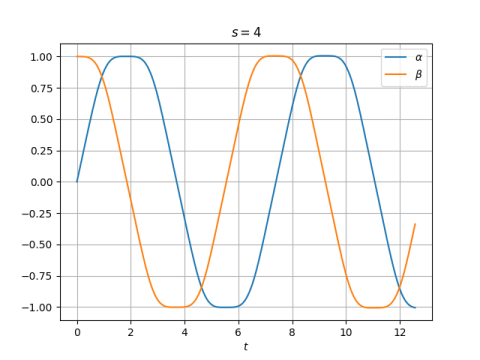The first zero of α(t) is at 3.7066, greater than π. In the plot t ranges from 0 to 4π, but the second period isn’t finished.

If we look at the phase plot, i.e (α(t), β(t)), we get a shape that I’ve blogged about before: a squircle!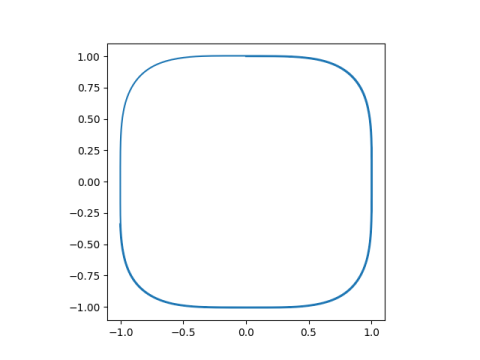This is because, as Shelupsky proved,## Odd order

The comments above mostly concern the case of even s. When s is odd, functions αs and βs don’t seem much like sine or cosine. Here are plots for s = 3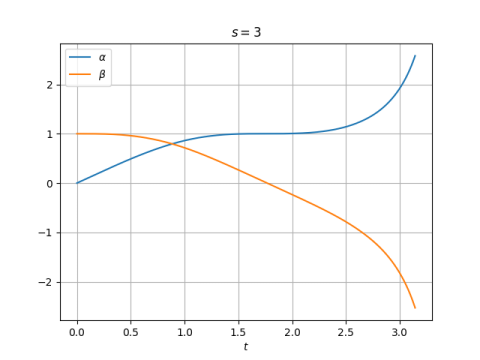and s = 5.## Other generalizations of sine and cosine

 David Shelupsky. A Generalization of the Trigonometric Functions. The American Mathematical Monthly, Dec. 1959, pp. 879-884

 After doing my numerical experiments I looked back more carefully at  and saw that the author proves that the solutions for even values of s are periodic, and that the periods increase with s, converging to 4 as s goes to infinity.

# Ripples and hyperbolas

I ran across a paper  this morning on the differential equation

y‘ = sin(xy).

The authors recommend having students explore numerical solutions to this equation and discover theorems about its solutions.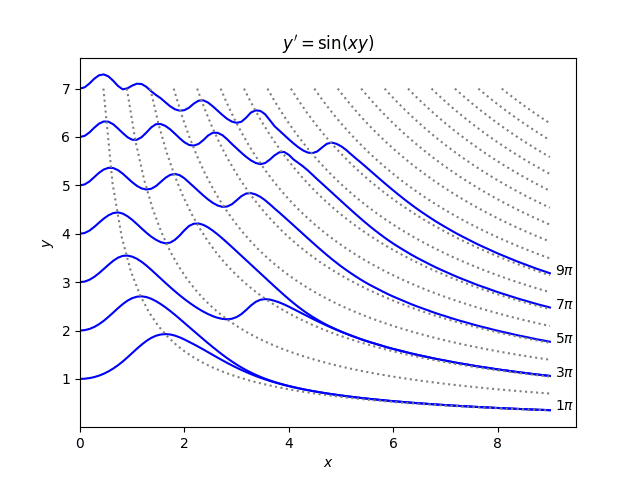Their paper gives numerous theorems relating solutions and the hyperbolas xy = a: how many times a solution crosses a hyperbola, at what angle, under what conditions a solution can be tangent to a hyperbola, etc.

The plot above is based on a plot in the original paper, but easier to read. It wasn’t so easy to make nice plots 40 years ago. In the original plot the solutions and the asymptotes were plotted with the same thickness and color, making them hard to tell apart.

## More differential equation posts

 Wendell Mills, Boris Weisfeiler and Allan M. Krall. Discovering Theorems with a Computer: The Case of y‘ = sin(xy). The American Mathematical Monthly, Nov., 1979, Vol. 86, No. 9 (Nov., 1979), pp. 733-739

# Driving vibrations with sawtooth waves

The previous post looked at driving a vibrating system with square waves rather than the more customary sine waves.

You could think of a square wave as a crude approximation to a sine wave. A sawtooth wave is another crude approximation to a sine wave, and so it would be interesting to see how systems driven by a sawtooth forcing function differ from those driven by a square or sinusoidal forcing function.With a square wave, the differences were most pronounced at low frequencies, i.e. when the driving frequency was low relative to the natural frequency. The same is true for sawtooth waves, but with some interesting differences.

As before we will look at the equation

u'' + u = sin(ωt)

and with sine replaced with a variation, this time a sawtooth wave rather than a square. We will use initial conditions u(0) = u‘(0) = 1.

We start with ω = 1, i.e. driving the system at its natural frequency.We see the resonance we’d expect when driving a system at its natural frequency. The amplitudes are lower for the sawtooth forcing function than the sinusoidal forcing function.

When we back away a little from the resonant frequency, setting ω = 0.9, we see beats.When we reduce ω to 0.5, we get resonance again for the sawtooth forcing solutions.At ω = 0.4 we see something like beats again.And at ω = 1/3 it looks like we have resonance again for the sawtooth-driven system.We also see beats at ω = 1/n for all integer n. I’ve tried this for n up to 12. But at other frequencies that are not reciprocals of integers I see periodic solutions.

I have only investigated this numerically. Do any of you know of analytical results that apply here?

Update: There’s a simple reason why driving frequencies of 1/n do indeed create resonance: The Fourier series for the driving function has a component at the resonant frequency. Thanks to gmvh for pointing this out.

The square wave has a similar resonance at driving frequency 1/n, but only when n is an odd number. I missed that because I didn’t happen to try any frequencies of that form. Here’s an example with n = 5.